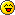Guest
Guest

##Gifts of the Traveler

on Tue Sep 14, 2010 10:06 am
So I always end up losing money when I buy Gifts of the Traveler, so I tried to figure out why. I used the drop rate research found on Guild Wars Wiki, I forget which. I multiplied the percentage chance of the drop by the value of the drop. I estimated the value of the drops, so comment if you think I estimated anything wrong.
10 Aged Hunter's Ales = 3000g x 0.0501 = 150.3
10 Hunter's Ales = 1000g x 0.0505 = 50.1
Keg of Aged Hunter's Ale = 13000g x 0.033 = 429
10 Champagne Poppers = 1500g x 0.0486 = 72.9
10 Bottle Rockets = 1500g x 0.049 = 73.5
10 Sparklers = 1500g x 0.0511 = 76.65
5 Crates of Fireworks = 5000g x 0.032 = 160
3 Disco Balls = 3000g x 0.0298 = 89.4
10 Jars of Honey = 3000g x 0.0477 = 143.1
10 Honeycombs = 3000g x 0.0467 = 140.1
5 Green Rock Candies = 2500g x 0.0492 = 123
5 Blue Rock Candies = 5000g x 0.0505 = 252.5
5 Red Rock Candies = 10000g x 0.0503 = 503
5 Mysterious Summoning Stones = 2500g x 0.15.31 = 382.75
5 Mercantile Summoning Stone = 2500g x 0.0557 = 139.25
5 Mysterious Tonics = 2500g x 0.1397 = 488.95
5 Cottontail Tonics = 2500g x 0.0474 = 118.5
Everlasting Cottontail Tonic = 280000g x 0.0006 = 168
Miniature Gwen Doll = 560000g x 0.0003 = 168
Miniature Proffesor Yakkington = 350000g x 0.0005 = 175
Miniature Brown Rabbit = 50000g x 0.0004 = 20
Vampiric Dragon Sword = 15000g x 0.002 = 30
Traveler's Bo Staff = 15000g x 0.0029 = 43.5
Traveler's Walking Stick = 15000g x 0.0019 = 28.5
Icy Dragon Sword = 15000g x 0.002 = 30
Ascalon Razor = 15000g x 0.0018 = 27
Moss Spider Egg = 30000g x 0.0025 = 75
Everlasting Crate of Fireworks = 500000g x 0.0005 = 250
150.3 + 50.1 + 429 + 72.9 + 73.5 + 76.65 + 160+ 89.4 + 143.1 + 140.1 + 123 + 252.5 + 503 + 382.75 + 139.25 + 488.95 + 118.5 + 168 + 168 + 175 + 20 + 30 + 43.5 + 28.5 + 30 + 27 + 75 250 = 4408
On average, from a Gift of the Traveler, your reward will be worth 4408g. I suggest not buying them for more than that.Valynn LionhartPosts : 1110
Location : Calgary, Alberta##Re: Gifts of the Traveler

on Tue Sep 14, 2010 11:18 am
Well, math is not my forte in the least, and this looks amazing to me. Perhaps Dylan or Scott are better suited to let you know if the math is right.Guest
Guest

##Re: Gifts of the Traveler

on Tue Sep 14, 2010 12:40 pm
I'm pretty sure my math is right, but I'm not sure my estimation of the prices is.SALPosts : 324##Re: Gifts of the Traveler

on Tue Sep 14, 2010 2:09 pm
It looks like it's probably fine to me, though I don't feel like going through the entire mathematical formula myself. The thing about gifts of the traveller is that it's a crap shoot, and even with a reliable formula you're not necessarily going to get that return on them, no matter how many you buy/open. Still, it seems like a reasonable way of pricing what the presents themselves should cost to buy or sell, I guess.

_________________
My Profile | My Characters | My Builds | JoJo